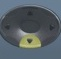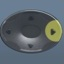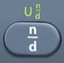## Sphere

##### Sphere = 3-Dimensional Round Shape with All Surface Points Equidistant from CenterBasics
r = radius = distance from surface to center
d = diameter = distance all the way across sphere through its center
SA = Surface Area = area covering surface of sphere (measured in square units)
V = Volume = space occupied by sphere (measured in cubic units)

Formulas (provided by Mathematics Formula Sheet)

SA = 4πr²

V =$\bf\displaystyle\frac{4}{3}$πr³

Formulas (NOT provided by Mathematics Formula Sheet)$\bf\displaystyle r=\frac{1}{2}d$

Question
A ball has a radius of 7 centimeters.  What is its surface area?

196π square centimeters

SA = 4πr²
SA = 4 × π × 7² = 196π

Calculator Click What You See Commentblinker clears screen
4 × π × 74*π*7² SA = 4πr²196π Answer
###### Sphere

Question
With reference to the question above, what is the area of the ball in decimal form?

615.7521601 square centimeters

See Toggle.
196π ⇔ 615.7521601

Calculator Click What You See Commentblinker clears screen
4 × π × 74*π*7² SA = 4πr²196π615.7521601 Answer
(Toggle)
###### Sphere

Question

With reference to the question above, what is the area of the sphere to the nearest tenth?

615.8 square centimeters

See Rounding.
615.7521601  615.8

Question
A ball has a diameter of$\bf\displaystyle14\frac{1}{2}$ centimeters.  To the nearest tenth of a square centimeter, what is its surface area?

660.5 square centimeters$\bf\displaystyle r=\frac{1}{2}d$$\bf\displaystyle r=\frac{1}{2}$*$\bf\displaystyle 14\frac{1}{2}$$\bf\displaystyle\frac{29}{4}$

A = 4πr²

A = 4*π*$\bf\displaystyle{{\frac{29}{4}}^{2}}$$\bf\displaystyle\frac{841\pi }{4}$ = 660.5198554 after toggle = 615.8 after rounding

Calculator Click What You See Commentblinker clears screen12×1412$\bf\displaystyle\frac{1}{2}$*$\bf\displaystyle 14\frac{1}{2}$$\bf\displaystyle r=\frac{1}{2}d$$\bf\displaystyle\frac{29}{4}$ r
4 × π ×2944*π*$\bf\displaystyle{{\frac{29}{4}}^{2}}$ SA = 4πr²$\bf\displaystyle\frac{841\pi }{4}$660.5198554 Answer
(before rounding)
###### Sphere

Question
A ball has a radius of 7 centimeters.
To the nearest hundredth of a cubic centimeter, what is it’s volume?

1436.76 cubic centimeters

V =$\bf\displaystyle\frac{4}{3}$πr³

V =$\bf\displaystyle\frac{4}{3}$*π*$\bf\displaystyle{{7}^{3}}$$\bf\displaystyle\frac{1372\pi }{3}$ = 1436.75504 after toggle = 1436.76 after rounding

Calculator Click What You See Commentblinker clears screen43× π ×3$\bf\displaystyle\frac{4}{3}$*π*$\bf\displaystyle{{7}^{3}}$ V =$\bf\displaystyle\frac{4}{3}$πr³$\bf\displaystyle\frac{1372\pi }{3}$1436.75504 Answer
(before rounding)
###### Sphere

Question
A ball has a diameter of$\bf\displaystyle14\frac{1}{2}$ centimeters.  To the nearest hundredth of a cubic centimeter, what is it’s volume?

1596.26 cubic centimeters$\bf\displaystyle r=\frac{1}{2}d$$\bf\displaystyle r=\frac{1}{2}$*$\bf\displaystyle 14\frac{1}{2}$$\bf\displaystyle\frac{29}{4}$

V =$\bf\displaystyle\frac{4}{3}$*π*$\bf\displaystyle{{\frac{29}{4}}^{3}}$ =$\bf\displaystyle\frac{24389\pi }{48}$ =1596.256317 after toggle = 1596.26 after rounding

Calculator Click What You See Commentblinker clears screen12×1412$\bf\displaystyle\frac{1}{2}$*$\bf\displaystyle 14\frac{1}{2}$$\bf\displaystyle r=\frac{1}{2}d$$\bf\displaystyle\frac{29}{4}$ r43× π ×2943$\bf\displaystyle\frac{4}{3}$*π*$\bf\displaystyle{{\frac{29}{4}}^{3}}$ V =$\bf\displaystyle\frac{4}{3}$πr³$\bf\displaystyle\frac{24389\pi }{48}$1596.256317 Answer
(before rounding)
###### Sphere

Practice – Questions
1.   A model globe has a radius of 6 inches.  What is its surface area?

2.   With reference to Question 1, what is the globe’s surface area to the nearest tenth?

3.  A model globe has a diameter of$\bf\displaystyle12\frac{1}{4}$ inches.  To the nearest tenth of a square inch, what is its surface area?

4.  A model globe has a radius of 6 inches.  To the nearest hundredth of a cubic inch, what is its volume?

5.  A model globe has a diameter of$\bf\displaystyle12\frac{1}{4}$ inches.  To the nearest hundredth of a cubic inch, what is its volume?